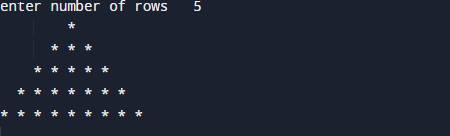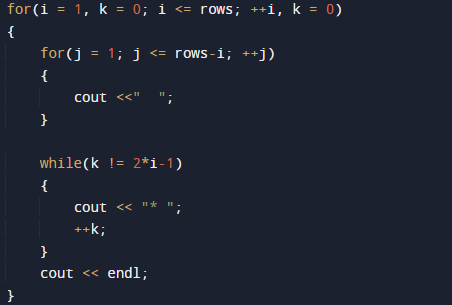# C++ Program to Creating a Pyramid

In this tutorial you will learn about the C++ Program to Creating a Pyramid and its application with practical example.

In this tutorial, we will learn to create a c++ program that will create Pyramid using c++ programming

## Prerequisites

Before starting with this tutorial we assume that you are best aware of the following C++ programming topics.

• if-else Statements.
• Looping statement.
• Basic input/output.
• Operators.

## What is Pyramid?

A Pyramid is a polygon structure and formed by connecting a polygonal base and a point. Each base edge and apex form a triangle.To print pyramid patterns in C++  we  will use  two nested for loops.The outermost loop is responsible for rows (*) of pyramid triangle where inner one  will print the required number of stars * in each row.

## C++ Program to Creating a Pyramid.

In this program we will print Pyramid using nested  for loop. We would first declared and initialized the required variables. Then we will create the Pyramid using below program.

## OutputIn the above program, we have first declared and initialized a set variables required in the program.

• i & j= for  iteration.
• rows = fro number of rows.
• k = for printing pattern.

In above program  first we declare variables i ,j ,k and rows , here we use 2 for loops.The outer loop will run for number of rows or pyramid and the inner loop print the number of stars to be displayed in each row.This is displayed using the following code.The outermost for loop here is responsible for the number of rows to be printed for star (*) pyramid triangle whereas inner one loop will print the required number of stars * pattern for each row.And the final output of our program is like that.

In this tutorial we have learn about the C++ Program to Creating a Pyramid and its application with practical example. I hope you will like this tutorial.